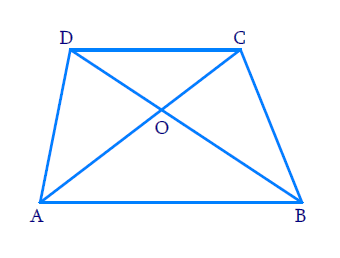# Ex.6.4 Q2 Triangles Solution - NCERT Maths Class 10

Go back to  'Ex.6.4'

## Question

Diagonals of a trapezium $$ABCD$$ with $$AB || DC$$ intersect each other at the point $$O.$$ If $$AB = 2 \,CD,$$ find the ratio of the areas of triangles $$AOB$$ and $$COD.$$

DiagramVideo Solution
Triangles
Ex 6.4 | Question 2

## Text Solution

Reasoning:

The ratio of the areas of two similar triangles is equal to the square of the ratio of their corresponding sides.

$$AA$$ criterion.

Steps:

In trapezium $$ABCD,$$  $$AB\parallel CD\,$$ and $$AB = 2\,CD$$

Diagonals $$AC, BD$$ intersect at ‘$$O$$

In $$\Delta AOB\,\text{and}\,\Delta COD$$

$$\angle AOB = \angle COD$$

(vertically opposite angles)
$$\angle ABO = \angle CDO$$

(alternate interior angles)
$$\Rightarrow \; \Delta AOB \sim \Delta COD$$

(AA criterion)
\begin{align}\Rightarrow \frac{{{\rm{Area \,of\,}}{{\Delta AOB}}}}{ \text{Area} \,of \Delta COD} & = \frac{{{{(AB)}^2}}}{{{{(CD)}^2}}} \\ & (\text{Theorem} \, 6.6) \end{align}

\begin{align} \qquad =\frac{{{{(2CD)}^2}}}{{{{(CD)}^2}}}&=\frac{{4C{D^2}}}{{C{D^2}}} = \frac{{4}}{1}\end{align}

\begin{align} \Rightarrow \; &\text{Area of } \Delta AOB : \text{Area of } \Delta COD \\=\;& 4:1 \end{align}

Learn from the best math teachers and top your exams

• Live one on one classroom and doubt clearing
• Practice worksheets in and after class for conceptual clarity
• Personalized curriculum to keep up with school